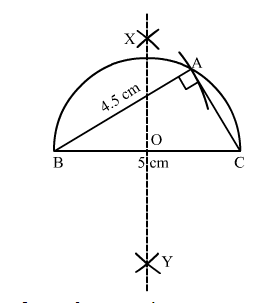# Construct a right-angled triangle whose hypotenuse measures 5 cm

Question:

Construct a right-angled triangle whose hypotenuse measures 5 cm and the length of one of whose sides containing the right angle measures 4.5 cm.

Solution:Steps of construction:
1. Draw a line segment BC = 5 cm.
2. Find the midpoint O of BC.
3. With O as the centre and radius OB, draw a semicircle on BC.
4. With B as the centre and radius equal to 4.5 cm, draw an arc cutting the semicircle at A.
4. Join AB and AC.
Thus, ABC is the required triangle.